# Indefinite Integral: Definition, Rules & Examples

Lesson Transcript
Instructor: Shaun Ault

Shaun is currently an Assistant Professor of Mathematics at Valdosta State University as well as an independent private tutor.

In this lesson, you will learn about the indefinite integral, which is really just the reverse of the derivative. We will discuss the definition, some rules and techniques for finding indefinite integrals, as well as a few examples.

## Definition

Given a function f(x), the indefinite integral (or antiderivative) of f (x) is a function F(x) whose derivative is equal to f (x). This means that F ' (x) = f (x).

Let's introduce a more convenient notation to stand for this concept. This is where that funny-looking long S symbol comes in.That symbol, together with its partner, the differential (dx, dt, dy, or similar, depending on the variables in the problem), are instructions for finding the indefinite integral (antiderivative) of a function f.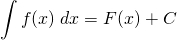The C is called the constant of integration. It is just an undetermined constant that gets tacked on to the antiderivative. But why? You might remember from the rules of differentiation that the derivative of any constant is simply 0. This is why we write + C because there is no way of knowing ahead of time what the constant term in the original function really was.

Now if the definition doesn't seem clear yet, don't worry. In the next few sections, we will see what this all means. First, a motivating example.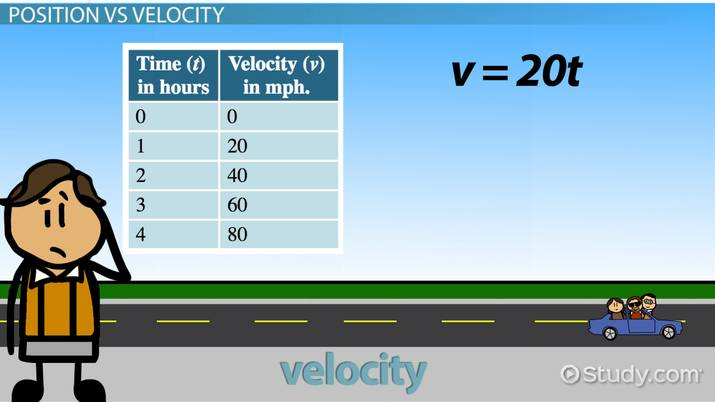An error occurred trying to load this video.

Try refreshing the page, or contact customer support.

Coming up next: Ordered Pair: Definition & Examples

### You're on a roll. Keep up the good work!

Replay
Your next lesson will play in 10 seconds
• 0:00 Definition
• 1:11 Position Vs. Velocity
• 2:41 Antiderivative Rules
• 3:31 Examples
• 4:44 Lesson Summary
Save Save

Want to watch this again later?

Timeline
Autoplay
Autoplay
Speed Speed

## Position vs. Velocity

Let's suppose you take a trip in your car, and your speed (or velocity) is known. Maybe your friend in the passenger seat has been looking at your speedometer and has written down the speed every hour for a total of four hours.

Time (t), in hours Velocity (v), in mph.
0 0
1 20
2 40
3 60
4 80

Based on the table, it seems like v = 20t mph. Can we figure out how far we've traveled? Sure we can: with calculus! More specifically, we will use an indefinite integral.

The key calculus concept is that velocity is the derivative of position. That is, v(t) = s' (t). So s is an antiderivative of v. Can you guess a function s(t) whose derivative will be 20t? If you guessed s = 10t^2, then pat yourself on the back.

Technically, we should also include the constant of integration, so the most general way we could express the position function s(t) would be: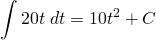However, we can safely ignore the C for a problem like this because we are only interested in the change in position.

Now, let's answer the original question: How far did you and your friend travel? Let's keep track of position by plugging the corresponding t value into the position function s(t).

Time (t), in hours Velocity (v = 20t), in mph. Position (s = 10t^2), in miles
0 0 0
1 20 10
2 40 40
3 60 90
4 80 160

The trip lasted t = 4 hours, so the total distance was s = 10(4)^2 = 160 miles.

## Antiderivative Rules

Finding antiderivatives in general is a tricky business. However, certain rules can guide us along. Two of the most important rules are the constant multiple rule and the power rule for antiderivatives.

The constant multiple rule states that any constant multiple may be pulled out of the integral, while the power rule tells us how to integrate expressions that are powers of the variable, such as x^2, t^3, and z^(-1/2).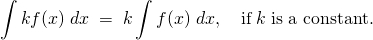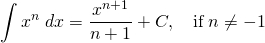To unlock this lesson you must be a Study.com Member.

### Register to view this lesson

Are you a student or a teacher?